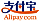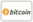Bookcover of Chaotic Oscillation of the Wave Equation and the Klein-Gordon Equation
Booktitle:

# Chaotic Oscillation of the Wave Equation and the Klein-Gordon Equation

LAP LAMBERT Academic Publishing (2016-04-13 )eligible for voucher
ISBN-13:

### 978-3-659-86170-3

ISBN-10:
3659861707
EAN:
9783659861703
Book language:
English
Blurb/Shorttext:
This book is written to review Goong Chen’s work, and to introduce some new work on the chaotic oscillations of the 1-D wave equation and the Klein-Gordon equation proposed by the author. It consists of three charpters: Charpter 1 introduces the chaotic oscillations of the 1D wave equation due to a self-excitation boundary codition, Charpter 2 concentrates on chaotic oscillations of the Klein-Gordon equation due to imbalance of distributed and boundary energy flows, Charpter 3 treats the Klein-Gordon equation with time-varying coefficients, and attempts to prove more bifurcations and chaos behaviors. In summary, the object of this book is to provide an introduction to the nonlinear phenomena of bifurcation and chaos in the wave equations and the Klein-Gordon equations.
Publishing house:
Website:
https://www.lap-publishing.com/
By (author) :
Bo Sun
Number of pages:
88
Published on:
2016-04-13
Stock:
Available
Category:
Mathematics
Price:
28.90 €
Keywords:
Klein-Gordon Equation, wave equation, chaotic oscillation, van der Pol boundary excitation### Categories